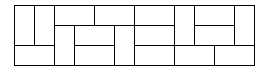## Wednesday, March 2, 2011

### poj_2663_Tri_Tiling.cpp

poj2663, uva10918, spoj3883, hit2124,

## Tri Tiling

 Time Limit: 1000MS Memory Limit: 65536K Total Submissions: 5244 Accepted: 2794
Description
In how many ways can you tile a 3xn rectangle with 2x1 dominoes?

Here is a sample tiling of a 3x12 rectangle.Input

Input
consists of several test cases followed by a line containing -1. Each
test case is a line containing an integer 0 <= n <= 30.
Output
For each test case, output one integer number giving the number of possible tilings.
Sample Input
```2
8
12
-1```
Sample Output
```3
153
2131```
Source
Waterloo local 2005.09.24

## Solution:

From the diagram we can get:
```T(n) = 3*T(n-2) + 2*T(n-4) + 2*(n-6) + ... + 2*T(2)
T(n-2) = 3*T(n-4) + 2*T(n-6) + 2*(n-8) + ... + 2*T(2)
Then, T(n) = 4T(n-2) - T(n-4)
if n is odd, then T(n) = 0;
if n is 0, T(n) = 1, since T(4) = 4T(2) - T(0), and T(4) = 11;
```

## Source Code:

```
//Wed Mar  2 13:30:14 CST 2011
#include <vector>
#include <list>
#include <map>
#include <set>
#include <deque>
#include <queue>
#include <stack>
#include <bitset>
#include <algorithm>
#include <functional>
#include <numeric>
#include <utility>
#include <sstream>
#include <iostream>
#include <iomanip>
#include <cstdio>
#include <cmath>
#include <cstdlib>
#include <cctype>
#include <string>
#include <cstring>
#include <cstdio>
#include <cmath>
#include <cstdlib>
#include <ctime>

using namespace std;

int check(int n) {
if (n == 0)return 1;
if (n == 2)return 3;
if (n & 1) return 0;
return 4 * check(n - 2) - check(n - 4);
}

int main(int argc, const char* argv[]) {
//freopen("input.in", "r", stdin);
//freopen("output.out", "w", stdout);
int n;
while (cin >> n && n != -1) {
cout << check(n) << endl;
}
//fclose(stdin);
//fclose(stdout);
return 0;
}

```